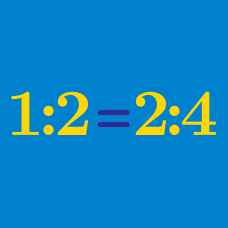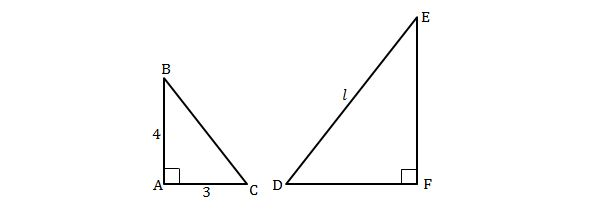Everyday Math

# Direct Variation

A worker can harvest all the corn in $9 \text{ m}^2$ of farmland in $1/3$ hours. How many hours will the worker need to harvest all the corn in $432 \text{ m}^2$ of farmland?

Gift set A contains $8$ red golf balls and $2$ white golf balls, and gift set B contains $5$ green golf balls and $3$ white golf balls. Suppose that Tom has some gift sets A and Judy has some gift sets B, and that each person has the same number of golf balls. Then what is the fraction of white balls among all the golf balls the two persons have?Two right triangles $ABC$ and $DEF$ in the above figure are similar, where the corresponding side of $\overline{AB}$ is $\overline{EF}.$ If $\lvert{\overline{AC}}\rvert=3,$ $\lvert{\overline{AB}}\rvert=4$ and $\lvert{\overline{DE}}\rvert=l=18,$ what is the length of $\overline{EF}?$

The value of $y$ changes directly proportional to the value of $x.$ If $y=18$ when $x=6,$ what is the value of $y$ when $x=29.5 ?$

The value of $y$ varies directly with the square of $x.$ If $y=22$ when $x=11,$ what is the value of $y$ when $x=22 ?$

×